# Test: EMFT - Electrical Engineering (EE)

Test Description

## 25 Questions MCQ Test GATE Electrical Engineering (EE) 2023 Mock Test Series - Test: EMFT

Test: EMFT for Electrical Engineering (EE) 2023 is part of GATE Electrical Engineering (EE) 2023 Mock Test Series preparation. The Test: EMFT questions and answers have been prepared according to the Electrical Engineering (EE) exam syllabus.The Test: EMFT MCQs are made for Electrical Engineering (EE) 2023 Exam. Find important definitions, questions, notes, meanings, examples, exercises, MCQs and online tests for Test: EMFT below.
Solutions of Test: EMFT questions in English are available as part of our GATE Electrical Engineering (EE) 2023 Mock Test Series for Electrical Engineering (EE) & Test: EMFT solutions in Hindi for GATE Electrical Engineering (EE) 2023 Mock Test Series course. Download more important topics, notes, lectures and mock test series for Electrical Engineering (EE) Exam by signing up for free. Attempt Test: EMFT | 25 questions in 75 minutes | Mock test for Electrical Engineering (EE) preparation | Free important questions MCQ to study GATE Electrical Engineering (EE) 2023 Mock Test Series for Electrical Engineering (EE) Exam | Download free PDF with solutions
 1 Crore+ students have signed up on EduRev. Have you?
Test: EMFT - Question 1

### A long cylindrical conductor of Radius R carries a current i. The current density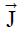is a function of radius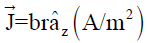, where b is constant. The Magnetic field H at any radial distance r will  be _____?

Detailed Solution for Test: EMFT - Question 1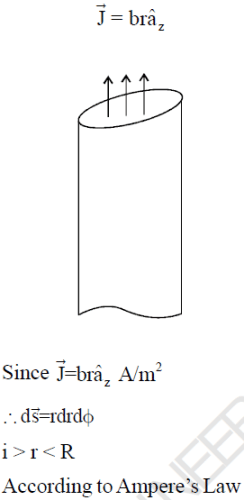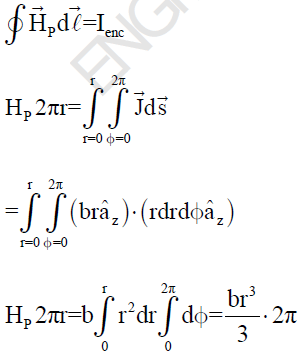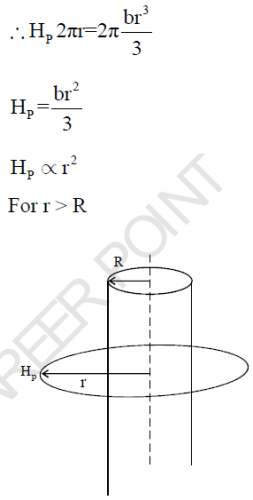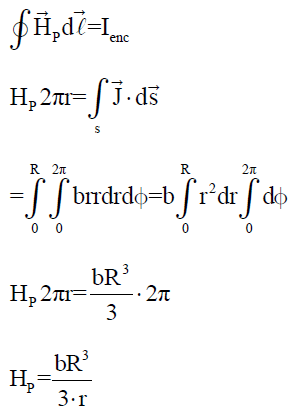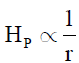Test: EMFT - Question 2

### The Magnetic vector potential obey which equation? 1.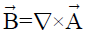2.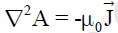3.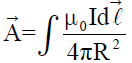Select the correct answer using the code given below

Test: EMFT - Question 3

### Plane defined by z = 0 carry surface current density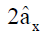A/m. The Magnetic intensity H is the two regions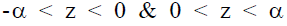are respectively?

Detailed Solution for Test: EMFT - Question 3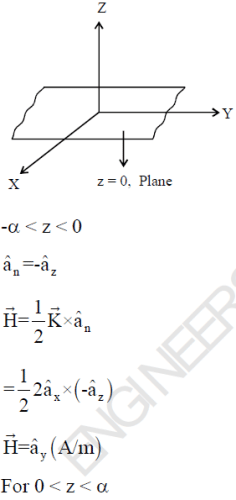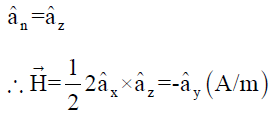Test: EMFT - Question 4

Two infinitely long wires separated by a distance 5m, carry currents I in opposite direction as shown in the
figure. If I = 8A, then the magnetic field intensity at point p is?Detailed Solution for Test: EMFT - Question 4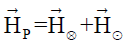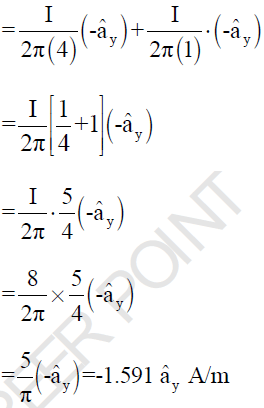Test: EMFT - Question 5

A current loop ABCD is held fixed on the plane of the paper as shown. The Magnetic field H at point ‘O’
will be ____?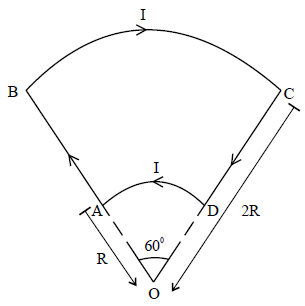Detailed Solution for Test: EMFT - Question 5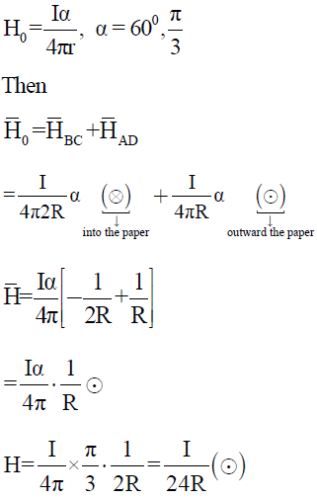Test: EMFT - Question 6

If A vector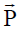is solenoidal, which of these is true?

Detailed Solution for Test: EMFT - Question 6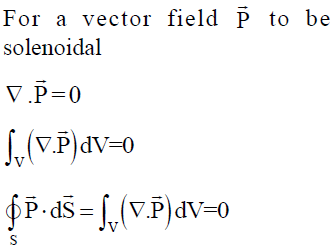Test: EMFT - Question 7

There is a region in which an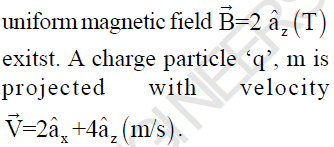The path followed by the charge particle will be____?

Detailed Solution for Test: EMFT - Question 7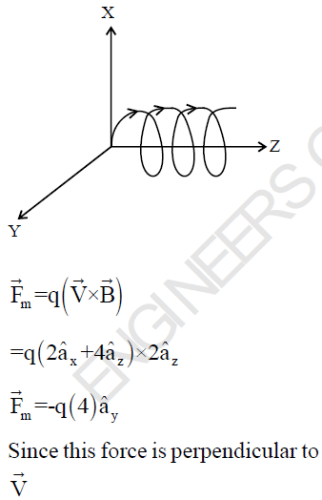Thus it under goes circular motion.
But z-component of velocity vector tends to move the charge particle in z-direction.
Hence path of charge particle is Helical.

Test: EMFT - Question 8

Which one of the following potentials does NOT satisfy Laplace’s Equation?

Detailed Solution for Test: EMFT - Question 8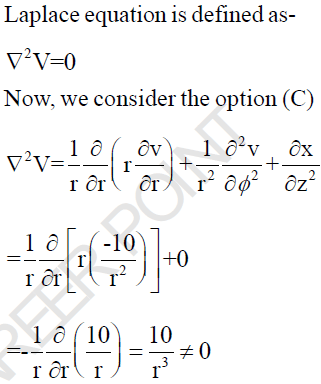Test: EMFT - Question 9

For the scalar field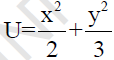, the magnitude of the gradiant at the point (1, 3) is?

Detailed Solution for Test: EMFT - Question 9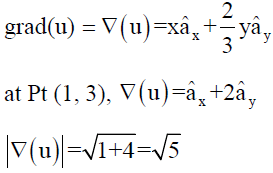Test: EMFT - Question 10

Given Scalar function f = xy + yz + zx. Find the Directional Derivative of ‘f’ at point (1, 2, 3) along the
vector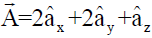Detailed Solution for Test: EMFT - Question 10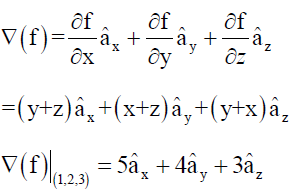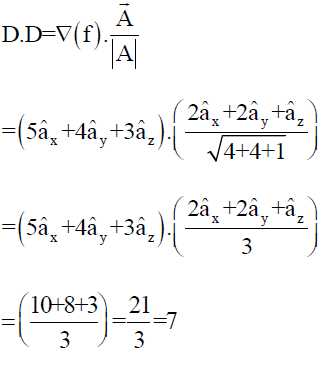Test: EMFT - Question 11

Which one of the following vector function has divergence & curl both zero.

Detailed Solution for Test: EMFT - Question 11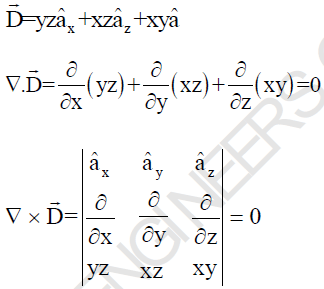Test: EMFT - Question 12

Which of the following vector can be expressed as curl of another vector

Detailed Solution for Test: EMFT - Question 12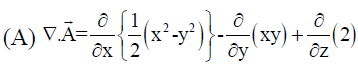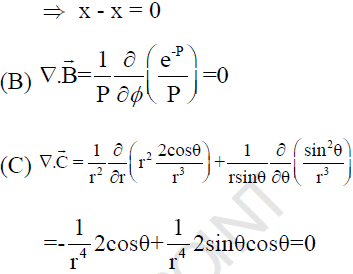So, The vector can be expressed as
curl of another vector.

Test: EMFT - Question 13

The expression for electric field in a space is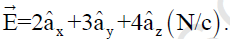Find the potential difference between point A (1, -2, 1) m and point B (2, 1, -2) m

Detailed Solution for Test: EMFT - Question 13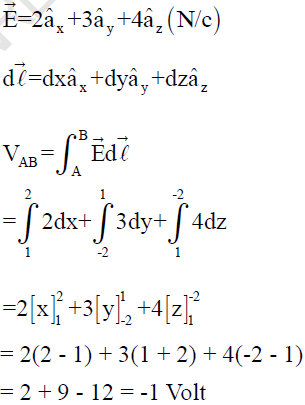*Answer can only contain numeric values
Test: EMFT - Question 14

Find the potential difference VAB between A (2, 1, 0)m & B (0, 2, 4)m in an electric field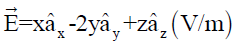in volts.

(Important - Enter the numerical value only in the answers)

Detailed Solution for Test: EMFT - Question 14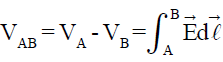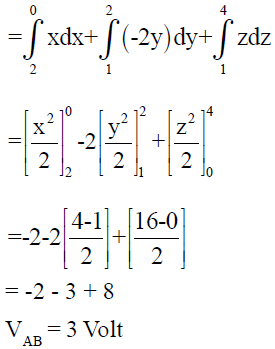Test: EMFT - Question 15

The force of attraction between the plate of parallel capacitor having charge q is____?

Detailed Solution for Test: EMFT - Question 15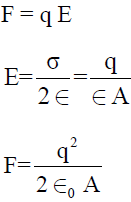Test: EMFT - Question 16

Four capacitors are arranged as shown. Find the amount of charge appear at 3F (between AB) capacitor?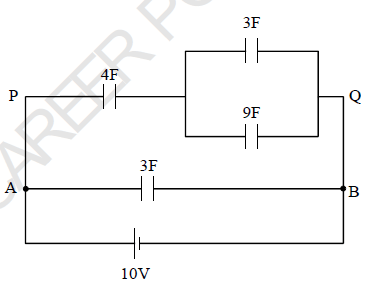Detailed Solution for Test: EMFT - Question 16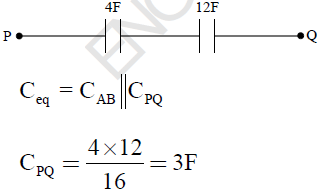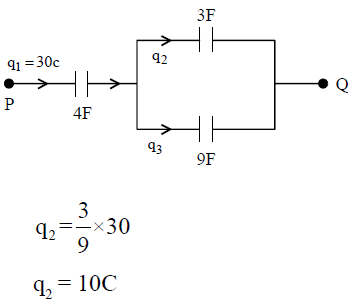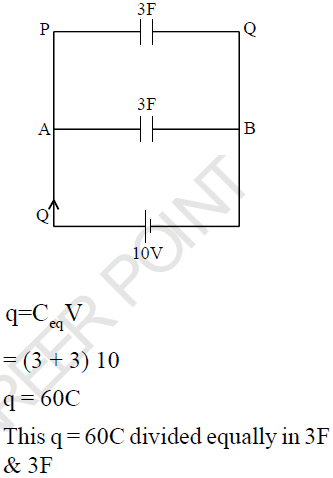Test: EMFT - Question 17

The current density in a cylindrical wire of radius 16mm placed along the z-axis is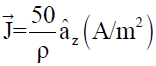. What will be the total current (in Ampere) flowing through the wire.

Detailed Solution for Test: EMFT - Question 17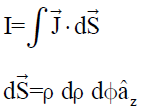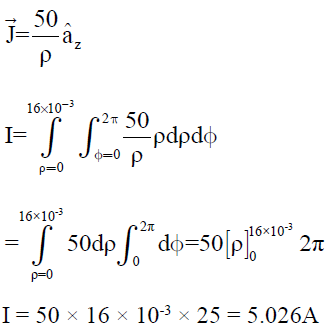Test: EMFT - Question 18

Two extensive homogeneous isotropic dielectrics meet on a plane z = 0. For z > 0,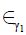= 4 and
for z < 0,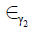= 2. A uniform electric field exists at z > 0 as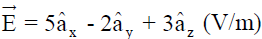. What is the value of electric field in the region z < 0

Detailed Solution for Test: EMFT - Question 18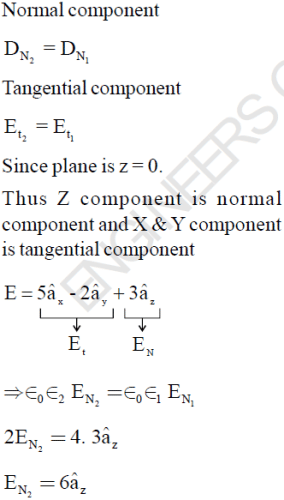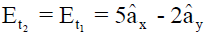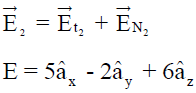Test: EMFT - Question 19

Find the voltage across the dielectric slab of dielectric constant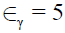when the voltage across the plate is 200V.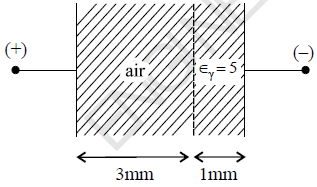Detailed Solution for Test: EMFT - Question 19

The combination becomes two capacitor in series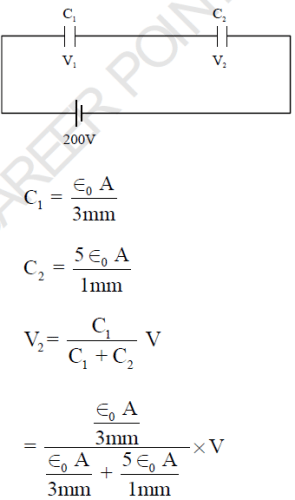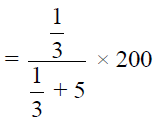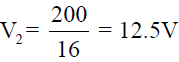Test: EMFT - Question 20

A Parallel plate capacitor is made of two circular plates separated by a distance of 5mm with a dielectric of dielectric constant 2.2 between them. When the electric field in the dielectric is 3×104 V/m, the charge density of the positive plate will be?

Detailed Solution for Test: EMFT - Question 20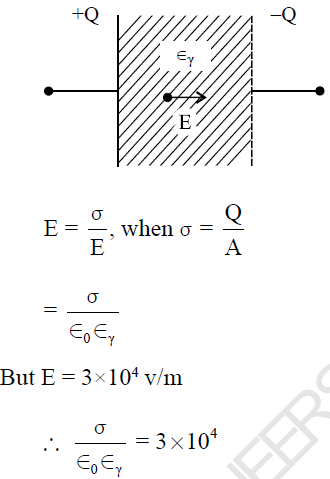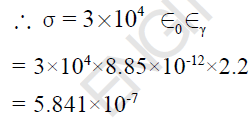Test: EMFT - Question 21

Consider the following diagram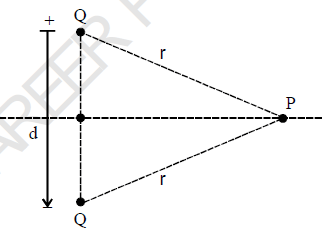The electric field E at a point P due to the Presence of dipole as shown in the above diagram (r >> d) is proportional to

Detailed Solution for Test: EMFT - Question 21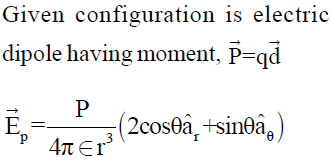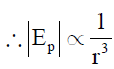Test: EMFT - Question 22

The potantial field V= 2/xyz  Valt. The vector form of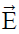at point (1, 1, 1) will be?

Detailed Solution for Test: EMFT - Question 22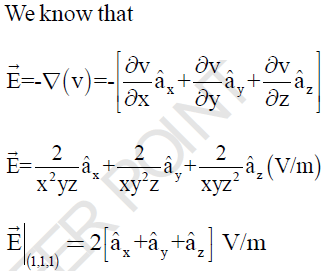Test: EMFT - Question 23

Consider the charge configuration and spherical gaussian surface as shown in the fig. When calculating the flux of the electric field oven the spherical gaussian surface will be due to the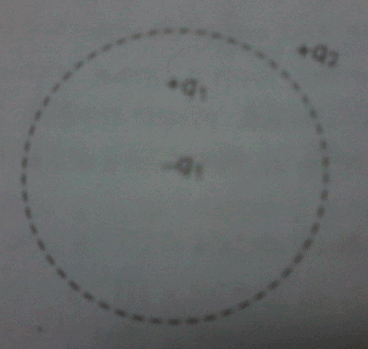Test: EMFT - Question 24

The electric potantial inside a charged spherical ball is given by v = ar2 + b, when r is the radial distance from centre a and b are constant. Then the charge density inside the ball will be?

Detailed Solution for Test: EMFT - Question 24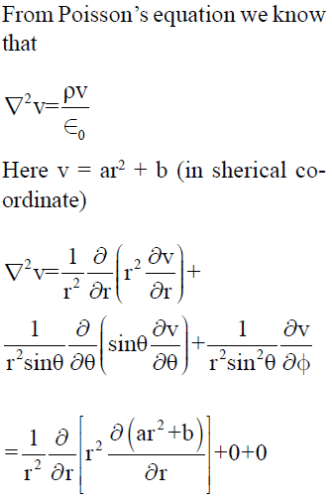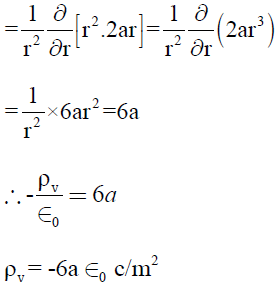Test: EMFT - Question 25

Two infinite sheet carry a charge density -4μ c/m2 & + 4μ c/m2 lies on y = +4 & y = -4 plane respectively. Find the electric field intensity in region defined by -4 < y < +4

Detailed Solution for Test: EMFT - Question 25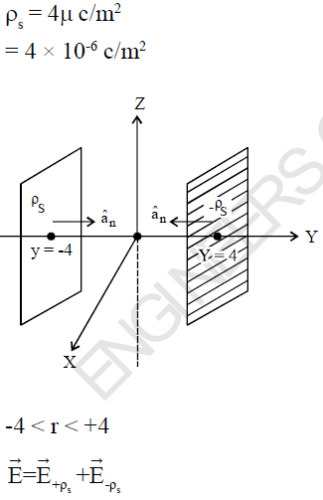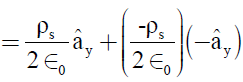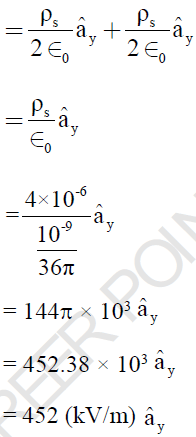## GATE Electrical Engineering (EE) 2023 Mock Test Series

22 docs|284 tests(Scan QR code)